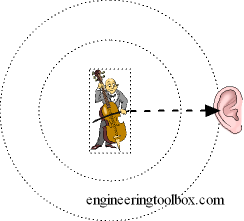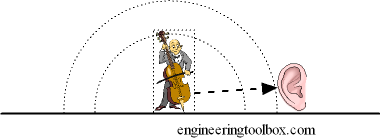Engineering ToolBox - Resources, Tools and Basic Information for Engineering and Design of Technical Applications!

# Sound - Abatement vs. the Distance from Source

## The disruption of the sound pressure wave and the reduction of noise is called attenuation - Sound Pressure Level vs. distance calculator.

The sound pressure from a source is reduced with distance from source.

### Spherical DistanceSound pressure in spherical distance from a noise source can be calculated as:

p2 = ρ c N / (4 π r2)              (1)

where

p = sound pressure (Pa, N/m2)

ρ = density of air (kg/m3)

c = speed of sound (m/s)

N = sound power (W)

π = 3.14

r = distance from source (m)

### Half Spherical DistanceSound pressure in half spherical distance from a source can be expressed as:

p2 = ρ c N / (4 π r2 / 2)

= 2 ρ c N / (4 π r2)                (2)

A more generic expression for sound pressure in distance from source can be formulated to:

p2 = D ρ c N / (4 π r2)              (3)

where

D = directivity coefficient (1 spherical, 2 half spherical)

The directivity coefficient depends on several parameters - the position and direction of the source, the room and the surrounding area, etc.

The Sound Pressure Level - Lp - can be expressed logarithmic in decibels as:

Lp = 20 log (p / pref)

= 20 log ((D ρ c N / (4 π r2))1/2 / pref)

= 20 log (1 / r (D ρ c N / (4 π))1/2 / pref)                 (4)

where

Lp = sound pressure level (dB)

pref = 2 10-5 - reference sound pressure (Pa)

Note! - a doubling of the distance from a sound source - will reduce the sound pressure level - Lp - with 6 decibels.

### Sound Pressure Level Calculator

ρ - air density (kg/m3)

c - sound velocity (m/s)

N - sound power (W)

r - distance from source (m)

### Example - Sound Pressure from a Wood Planer

The sound power generated from a wood planer is estimated to 0.01 W. The sound pressure in distance 10 m from the planner can be calculated as

Lp = 20 log ((D ρ c N / (4 π r2))1/2 / pref )

= 20 log (2 (1 kg/m3) (331.2 m/s) (0.01 W) / (4 π (10 m)2))1/2 / (2 10-5 Pa))

= 71 dB

## Related Topics

• Acoustics - Room acoustics and acoustic properties, decibel A, B and C, Noise Rating (NR) curves, sound transmission, sound pressure, sound intensity and sound attenuation.

## Engineering ToolBox - SketchUp Extension - Online 3D modeling!

Add standard and customized parametric components - like flange beams, lumbers, piping, stairs and more - to your Sketchup model with the Engineering ToolBox - SketchUp Extension - enabled for use with the amazing, fun and free SketchUp Make and SketchUp Pro .Add the Engineering ToolBox extension to your SketchUp from the SketchUp Pro Sketchup Extension Warehouse!

Translate

## Privacy

We don't collect information from our users. Only emails and answers are saved in our archive. Cookies are only used in the browser to improve user experience.

Some of our calculators and applications let you save application data to your local computer. These applications will - due to browser restrictions - send data between your browser and our server. We don't save this data.

## Citation

• Engineering ToolBox, (2005). Sound - Abatement vs. the Distance from Source . [online] Available at: https://www.engineeringtoolbox.com/abatement-distance-source-d_723.html [Accessed Day Mo. Year].

Modify access date.

. .

#### Scientific Online Calculator3 30